##Brocard pivoting

Consider a Brocard point J of the triangle ABC, characterized by the equality of angles ang(BAJ), ang(CBJ), ang(ACJ) (see Brocard.html ). Take a point D on BC and construct the circle c1 = {DBJ} cutting AB at E, then the circle c2 = {AEJ} cutting AC at F, then circle c3 = {FCJ}. The following facts are true and easy to prove:
(1) c3 passes through D.
(2) ang(JDB) = ang(JFC) = ang(JEA) and triangles JDB, JEA and JFB are similar.
(3) triangle DEF is similar to ABC for all positions of D on line BC.
(4) ang(BDF) = ang(CFE) = ang(AED).
(5) J is also a Brocard point of triangle DEF (ang(DEJ) = ang(FDJ) = ang(EFJ)).
(6) the ratios (|AD|:|BF|:|CE|) are equal to ((c/b):(a/c):(b/a)), trilinear coordinates of J. Later equality of ratios is a necessary and sufficient condition for three points D, E, F on the sides of ABC, to define a triangle DEF, which is similar to ABC and pivotal, with respect to the first Brocard point.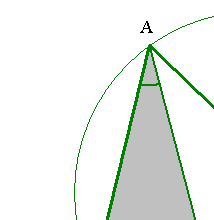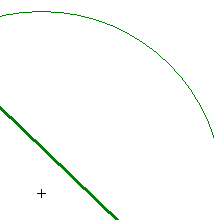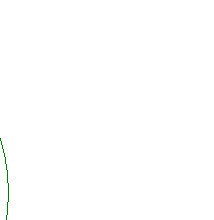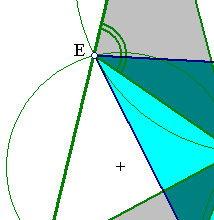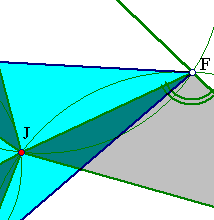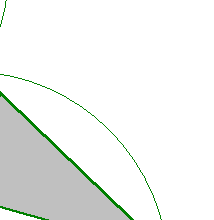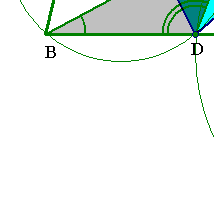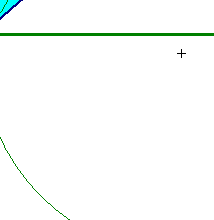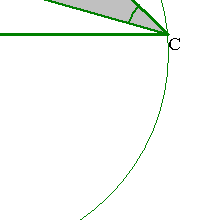For a nice application of the last (6) property on a particular pivotal triangle, related to the altitudes of ABC, look at the file Yagci.html .

 Produced with EucliDraw© http://users.math.uoc.gr/~pamfilos/